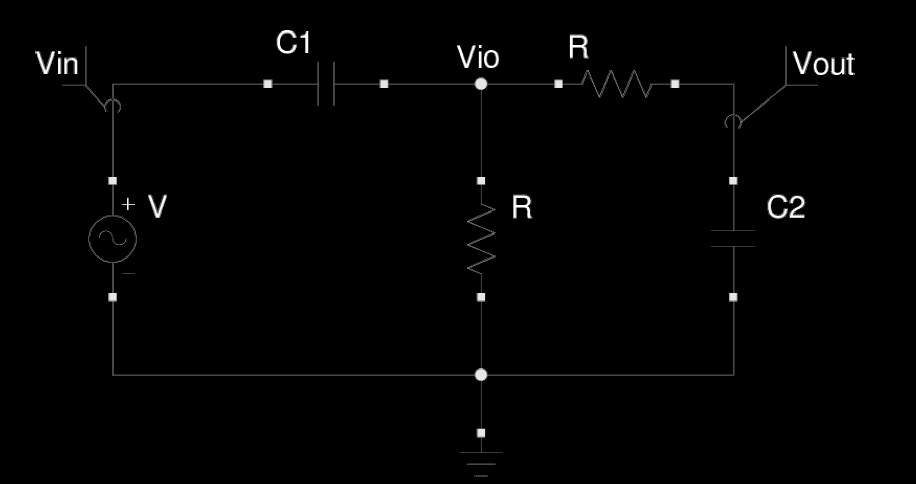# Find the Differential Equation of an Analog Band Pass Filter

I don't even know how to start. We're supposed to find the differential equations that links $V_{in}$, $V_{out}$, and $V_{io}$, as well as the values of $C_1$, $C_2$ and $R$ so the filter works in the audible spectrum. We're allowed to use Fourier transform, if that simplifies anything.I thought of splitting the band pass filter as a high pass at first, and a low pass after.

That way I can relate $V_{io}$ with $V_{in}$, and $V_{out}$, with $V_{io}$, in two different equations.

Doing that... the results were:

$RC \frac{\mathrm{d} Vin(t)}{\mathrm{d} t} = Vio(t) + RC \frac{\mathrm{d} Vio(t)}{\mathrm{d} t}$

for the high pass filter

$Vio(t) = Vout(t) + RC \frac{\mathrm{d} Vout(t)}{\mathrm{d} t}$

for the low pass filter

Then, using.. $fc = \frac{1}{2 \pi R C}$

For the audible frequency, it'd be between $20$ hz- $20$ KHZ. So using that, I should be able to express both $C_1$ and $C_2$ as a function of $R$.

However, how do I actually determinate the value of $R$ required? or do I assume a set value?

If there's anything wrong, please let me know why. Thank you, have a nice day.

• The answers below don't directly address your question. Do you know any of the circuit theory techniques? Loop analysis/signal flow analysis/or just writing down the loop voltage and node current conservation equations and reducing them; or Laplace transform although that is not what is asked for it can be reverse engineered. May 6 '15 at 11:46

I'm afraid your first equation is wrong because it assumes a highpass filter without any load. However, there is a lowpass filter connected at its output and for this reason the current through the resistor leading to ground does not equal the current through $C_1$.

What you need to do is apply the complex voltage divider rule in the frequency domain, which will give you

$$\frac{V_{io}(\omega)}{V_{in}(\omega)}=\frac{Z(\omega)}{1/j\omega C_1+Z(\omega)}\tag{1}$$

where $Z(\omega)$ is the impedance of $R$ in parallel with the series connection of $R$ and $C_2$. I'm sure you can figure this out. The relation between $V_{out}$ and $V_{io}$ is simply

$$\frac{V_{out}(\omega)}{V_{io}(\omega)}=\frac{1/j\omega C_2}{1/j\omega C_2+R}=\frac{1}{1+j\omega RC_2}\tag{2}$$

The total response $V_{out}/V_{in}$ is simply obtained by multiplying Eq. (1) with Eq. (2). The time domain differential equations are obtained by multiplying Eqs (1) and (2) by the denominators of both sides and noting that a multiplication by $j\omega$ corresponds to differentiation.

• Could it be that if the problem assumes that the circuit is not initially powered my solution would be correct? Or other condition like that. The assignment supposes we come up with an equation in the time domain, since we're asked to use fourier transformations later on to join them all together, and use the antitransform to come up with a single merged equation. Thank you very much for your response. May 5 '15 at 14:45
• @martin1982: No, it's not correct. You can do everything in the time domain, but it's easier the way I showed it. You can derive the time-domain equations as explained in the last sentence of my answer. May 5 '15 at 15:02

There's a problem in your solution — it doesn't take into consideration the influence of a LPF on HPF. (Matt L. already mentioned that)

The exact value of R (which you will base on while calculating $C_1$ and $C_2$) is usually chosen to produce the output resistance of a filter at least 10 times lower than a load resistance (connected to $V_{out}$) AND an input resistance at least 10 times higher than internal resistance of a signal source.